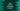In this post, we will learn what is method overloading in Java and how it works with examples.

A Java class can have multiple methods with the same name but different parameters and different return types. This is called method overloading and the method is called overloaded method.

We can change the number of arguments that method is accepting and also the data type of the arguments. The return type is also changeable. With method overloading, the readability of the program can be improved. Let me show you an example how method overloading works.

Let’s consider the below program:

``````class Util{
public static int getHalf(int number){
return number/2;
}
}

public class Main {
public static void main(String[] args) {
System.out.println(Util.getHalf(10));
}
}``````

In this program, the Util class has one static method getHalf that takes one integer number as its parameter and returns the integer value of half of that number.

But, if we want to find half of a floating point number, we can’t use this method. We can create another method with a different name, but instead of using a different name for the same use case, we can create another method with the same name but a different parameter type and return type.

``````class Util{
public static int getHalf(int number){
return number/2;
}

public static float getHalf(float number){
return number/2;
}
}

public class Main {
public static void main(String[] args) {
System.out.println(Util.getHalf(4));
System.out.println(Util.getHalf(11f));
}
}``````

I created a new method that takes one float number as its parameter and returns the result in float.

It will print:

``````2
5.5``````

We can use method overloading with a different number of parameters. The number of parameters can differ for the overloaded methods. For example,

``````class Log {
public static void debug(String message) {
System.out.println("Log: " + message);
}

public static void debug(String message, int error) {
System.out.println("Log: " + message + ", Error: " + error);
}
}

public class Main {
public static void main(String[] args) {
Log.debug("This is a debug message");
Log.debug("Error occurred!! ", 404);
}
}``````

In this example, we created a class Log with an overloaded method debug. We can either pass one string or one string with an integer error code to it.

If you run this program, it will print:

``````Log: This is a debug message
Log: Error occurred!! , Error: 404``````

As you can see here, we can have different body and parameters with the same method name for overloaded methods.

### Can we use method overloading with only change in return type:

We can’t use method overloading without changing anything in the parameters. Even if we change the return type, the compiler will throw an error. For example:

``````class Util{
public static int getHalf(int number){
return number/2;
}

public static float getHalf(int number){
return (float)number/2;
}
}``````

For this class, we are trying to overload the getHalf method with a change in the return type. But the compiler will throw an error:

It is showing an error that the method is already defined in the class.